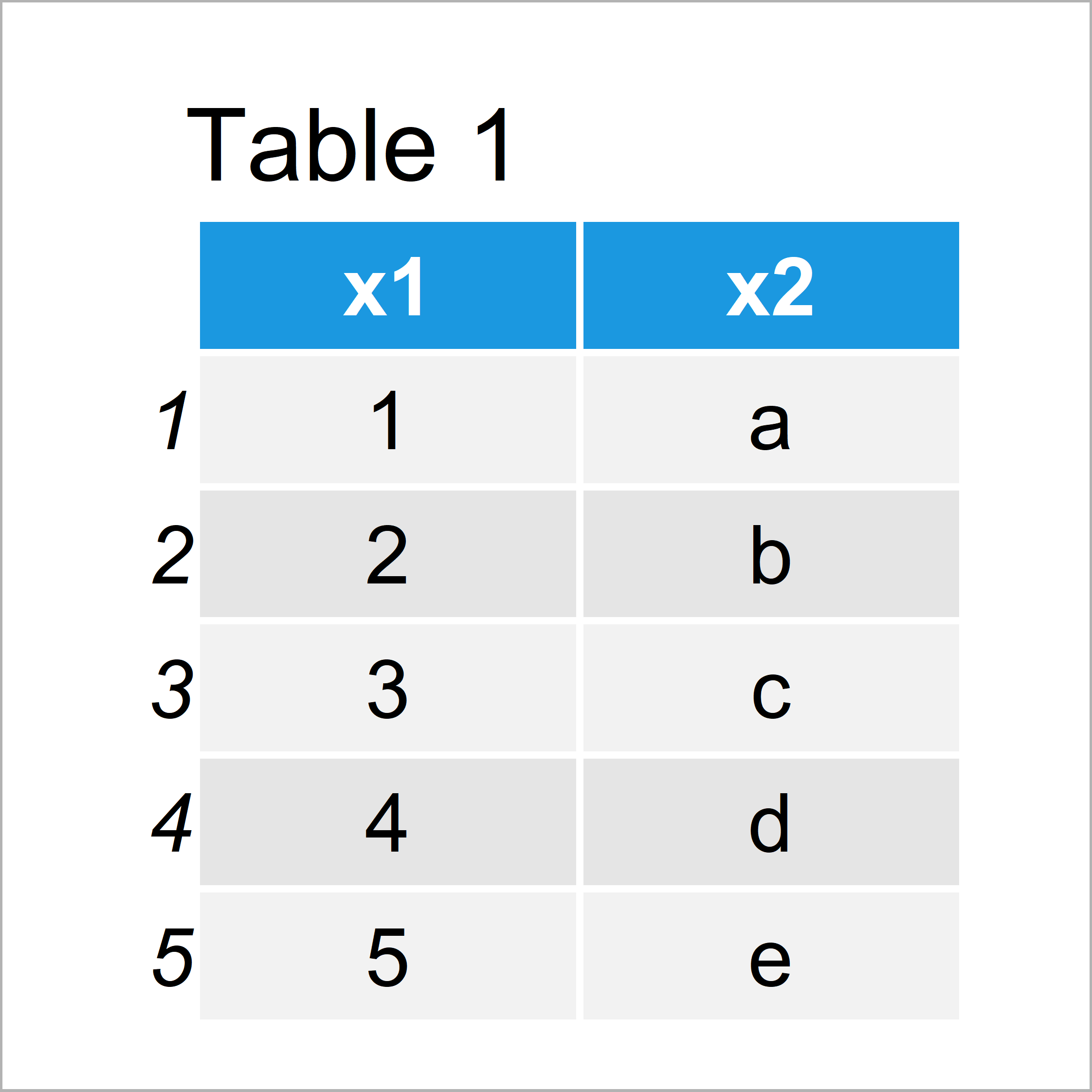# R attach Warning: The following objects are masked

In this R article you’ll learn how to deal with the attach function warning “The following objects are masked”.

The page is structured as follows:

Let’s start right away.

## Constructing Example Data

The following data will be used as basement for this R programming language tutorial.

```data1 <- data.frame(x1 = 1:5,      # Creating first data frame
x2 = letters[1:5])
data1                              # Printing first data frame```Table 1 shows the structure of the first example data – It contains five rows and two columns that are called x1 and x2.

Let’s create another data frame:

```data2 <- data.frame(x1 = 11:15,    # Creating second data frame
x2 = letters[11:15])
data2                              # Printing second data frame```After running the previous R code the data frame shown in Table 2 has been created. It also contains the variables x1 and x2. However, the values are different as in the first data frame.

## Example 1: Reproduce the Warning Message – The following objects are masked

Example 1 illustrates how to replicate the warning message “The following objects are masked” after executing the attach function in R.

Let’s first attach our first data frame:

`attach(data1)                      # Attaching first data frame`

Now, we can access the columns of this data frame without specifying the name of the data:

```x1                                 # Printing x1 of first data frame
#  1 2 3 4 5```

Now, let’s assume that we want to access the columns of the second data frame using the attach function:

```attach(data2)                      # Attaching second data frame
# The following objects are masked from data1:
#
#   x1, x2```

Damn, the RStudio console returned the warning “The following objects are masked”.

The reason for this is that we have not detached the first data frame before attaching the second data frame.

Since both data frames contain variables with the same name, the R programming language is not sure which variable you want to use.

Next, I’ll explain how to solve this problem.

## Example 2: Fix the Warning Message – The following objects are masked

This example explains how to avoid the warning “The following objects are masked”.

Before running the following R code, you may have to restart your R session to make sure no other data frames are attached.

Anyway, let’s begin the example with attaching the first data frame:

`attach(data1)                      # Attaching first data frame`

Now, we can print the values in one of the columns:

```x1                                 # Printing x1 of first data frame
#  1 2 3 4 5```

The next step is key: The following R code uses the detach function to detach our first data frame:

`detach(data1)                      # Detaching first data frame`

Now, we can attach another data frame without getting the warning message:

`attach(data2)                      # Attaching second data frame`

## Video, Further Resources & Summary

Do you want to learn more about attach and detach? Then I can recommend watching the following video of my YouTube channel. I illustrate the examples of this tutorial in the video:

Besides the video, you may want to have a look at the related posts at https://statisticsglobe.com/. You can find some tutorials about data frames below.

At this point you should know how to avoid the warning message “The following objects are masked” in the R programming language. Let me know in the comments, in case you have further questions and/or comments.

Subscribe to the Statistics Globe Newsletter

•James
February 3, 2023 9:44 pm

this didn’t solve the issues

•February 6, 2023 10:09 am

Hello James,

When did you get this error, and after which command? Could you please share your script with us?

Regards,
Cansu

•James
February 6, 2023 7:25 pm

attach(data)
plot(x, y)

and I get the following error:
The following objects are masked _by_ .GlobalEnv:

a, y

The following objects are masked from data (pos = 3):

a, b, x, y

The following object is masked from data (pos = 10):

y

The following object is masked from bacteria (pos = 13):

y

The following objects are masked from data_two (pos = 16):

a, b, x, y

The following objects are masked from data (pos = 21):

a, b, x, y

The following object is masked from data (pos = 30):

y

The following object is masked from bacteria (pos = 33):

y

However, I found that not using attach() was the way to go. I used the with() command for better results:
i.e.
with(data, plot(x, y))

•February 7, 2023 11:54 am